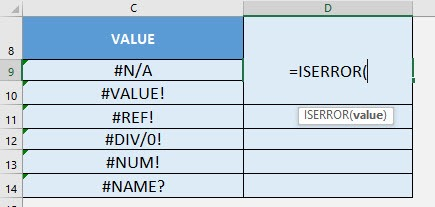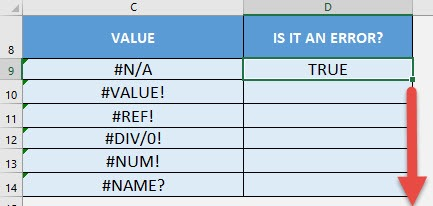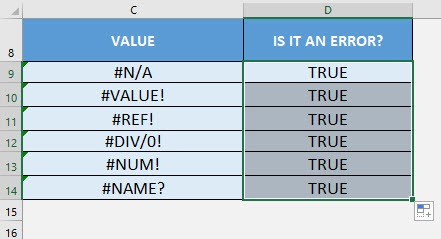What does it do?

Checks if the cell value is an error or not

Formula breakdown:

=ISERROR(value)

What it means:

=ISERROR(value to be checked if it is an error or not)

Have a bunch of values and you need to check which ones are errors? The ISERROR Formula in Excel is perfect for this!

There are a couple of interesting points to take note of the ISERROR Formula:

• The following are treated as errors by the ISERROR Formula
• #N/A
• #VALUE!
• #REF!
• #DIV/0!
• #NUM!
• #NAME?
• #NULL!

I explain how you can do this below:STEP 1: We need to enter the ISERROR function in a blank cell:

## =ISERROR(STEP 2: The ISERROR arguments:

## value

What is the value to be checked?

Select the cell containing the value you want to be checked if it is an error:

## =ISERROR(C9)Apply the same formula to the rest of the cells by dragging the lower right corner downwards.You can now see which ones are errors!How to Use the ISERROR Formula in Excel